# 每日算法系列【LeetCode 927】三等分

+关注继续查看

## 题目描述

• A, A, ..., A[i] 组成第一部分；
• A[i+1], A[i+2], ..., A[j-1] 作为第二部分；
• A[j], A[j+1], ..., A[A.length - 1] 是第三部分。
• 这三个部分所表示的二进制值相等。

输入：
[1,0,1,0,1]

[0,3]

输入：
[1,1,0,1,1]

[-1,-1]

• 3 <= A.length <= 30000
• A[i] == 0 或 A[i] == 1

## 代码

### c++

class Solution {
public:
vector<int> threeEqualParts(vector<int>& A) {
int n = A.size();
int cnt1 = accumulate(A.begin(), A.end(), 0);
if (cnt1 % 3) return {-1, -1};
if (!cnt1) return {0, 2};
cnt1 /= 3;
int cnt = 0, l, r;
for (int i = 0; i < n; ++i) {
if (!A[i]) continue;
cnt++;
if ((cnt-1)%cnt1 == 0) l[(cnt-1)/cnt1] = i;
if (cnt%cnt1 == 0) r[(cnt-1)/cnt1] = i;
}
for (int i = 0; i < 3; ++i) {
r[i] += n - 1 - r;
}
for (int i = 0; i <= r-l; ++i) {
if (A[l+i] != A[l+i] || A[l+i] != A[l+i])
return {-1, -1};
}
return {r, r+1};
}
};

### python

class Solution:
def threeEqualParts(self, A: List[int]) -> List[int]:
n = len(A)
cnt1 = sum(A)
if cnt1%3 != 0:
return [-1, -1]
if cnt1 == 0:
return [0, 2]
cnt1 //= 3
cnt = 0
l, r = *3, *3
for i in range(n):
if A[i] == 0:
continue
cnt += 1
if (cnt-1)%cnt1 == 0:
l[(cnt-1)//cnt1] = i
if cnt%cnt1 == 0:
r[(cnt-1)//cnt1] = i
for i in range(3):
r[i] += n - 1 - r
if A[l:r+1] != A[l:r+1] or
A[l:r+1] != A[l:r+1]:
return [-1, -1]
return [r, r+1]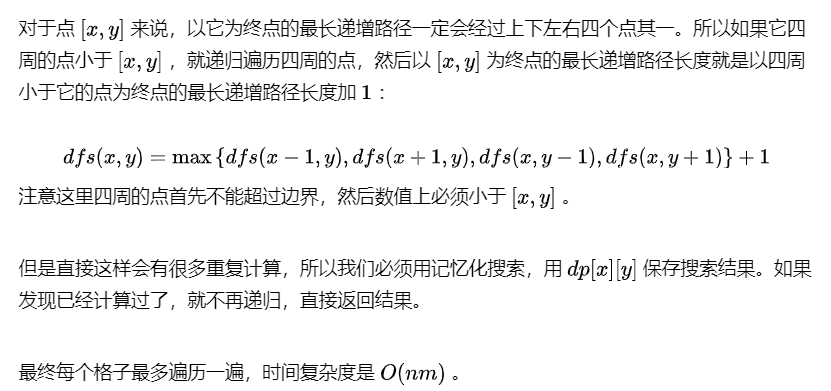20 0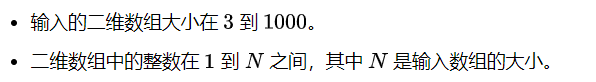13 0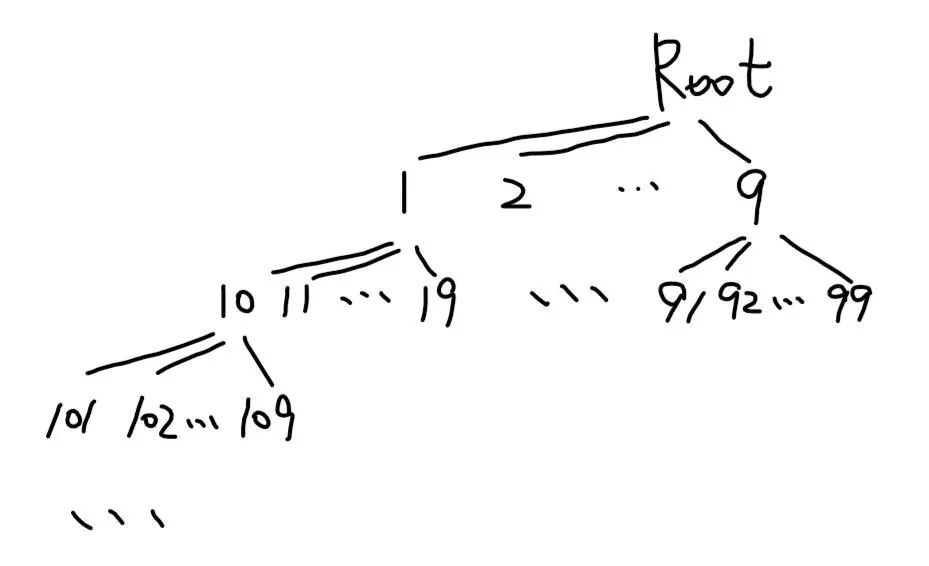26 0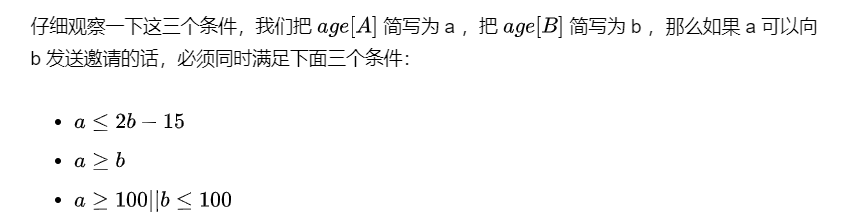21 0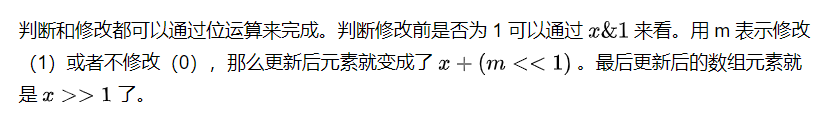15 0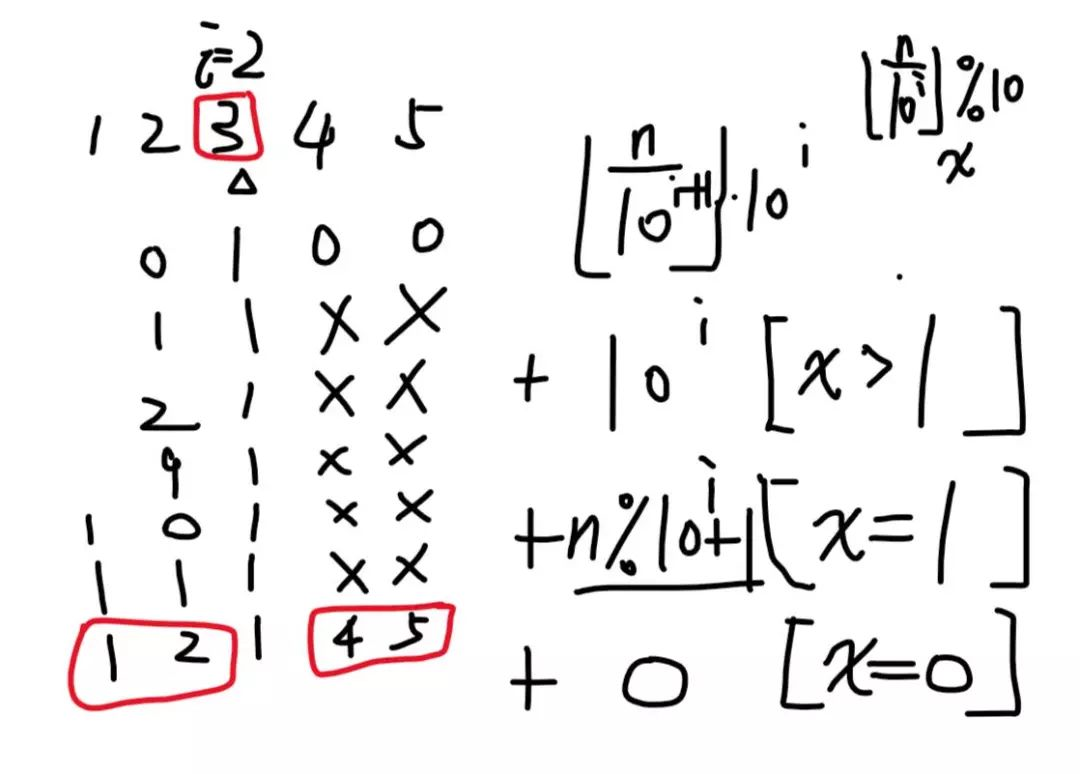27 022 0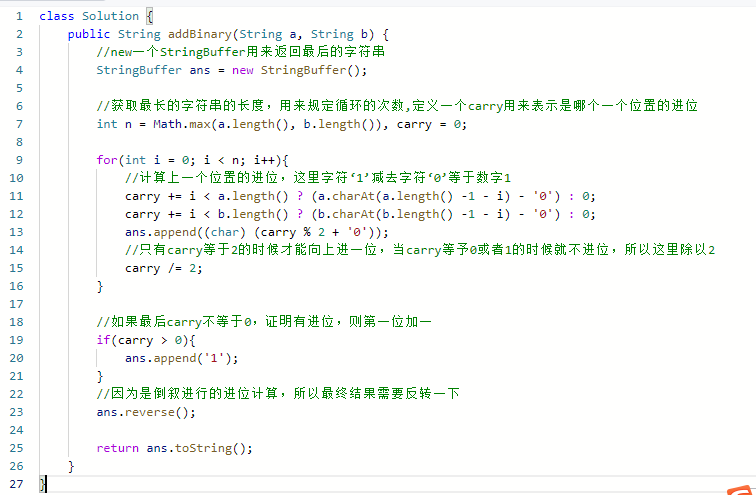leetcode算法67.二进制求和

23 0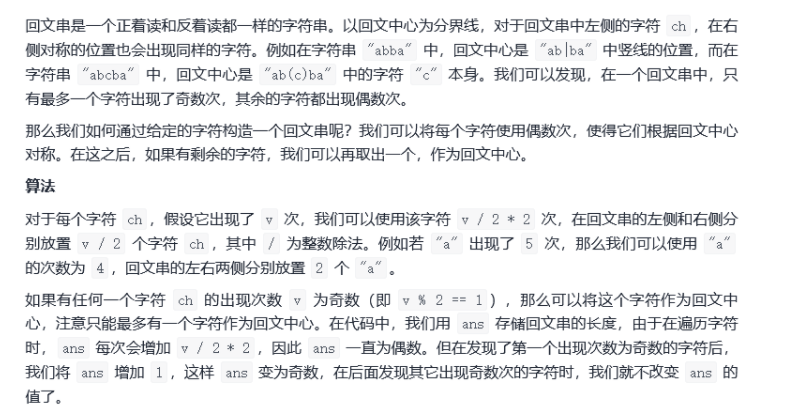【算法千题案例】每日LeetCode打卡——73.最长回文串
📢前言 🌲原题样例：最长回文串 🌻C#方法：排序遍历 🌻Java 方法一：计数 💬总结
58 0
217

0